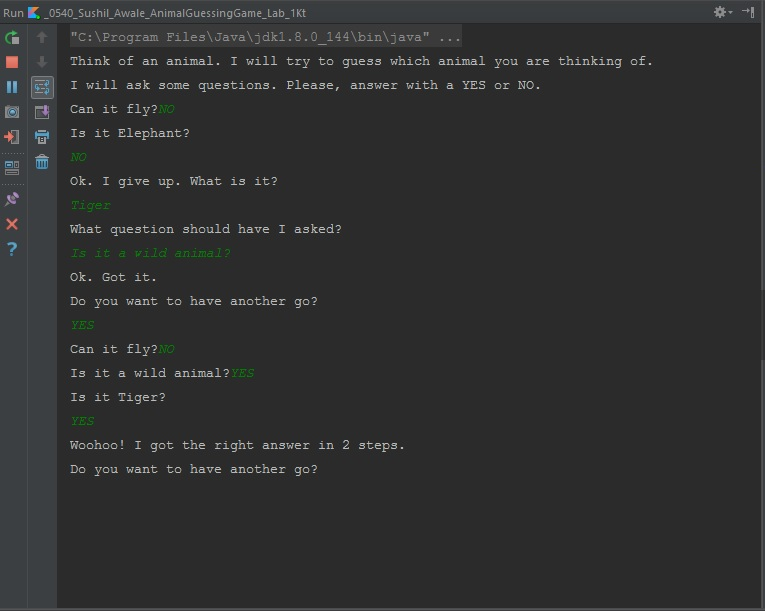Build an Animal Guessing Game in Kotlin

Animal Guessing Game is a toy problem in Artificial Intelligence and is one of the best introductory projects for programmers who want to learn AI. I am taking Artificial Intelligence course for my fifth semester and this is my first AI project which I wrote in Kotlin. Animal Guessing Game as the name suggests the guesses the animal that the player is thinking of.

Here is how the game goes:

## Description

First, the Animal Guessing Game agent asks the player to think of an animal. Then the agent will ask a series of Yes/No questions to narrow down its guess. When the agent runs out of questions to ask, it makes a guess and asks if it got is correct. If the agent got the answer correct, it wins. Otherwise, the agent asks the player what it was thinking of and also asks what question should the agent had asked to make the correct guess. In other words, the agent learns from the player so that it can make a better guess next time.

## Demo Snapshot

Here is a snapshot of a run in the game.## Understanding The Problem

Here, it is very important to understand the problem. This is a simple binary problem i.e. ask the player a Yes/No question; if the answer is ‘Yes’ go to this question; if the answer is ‘No’ go to that question. Hence, it is best implemented in a Binary Search Tree which I have used.

## Implementation

### Binary Search Tree

Create a simple Binary search tree [Wiki] with Nodes containing data (A Yes/No question or an animal name) and two children nodes. The binary search tree consists of a function to insert a child node which the agent uses to store new information.

### Animal Guessing Game Agent

Next, create the Animal Guessing Game Agent. It will contain the following functions:

• A function to start the game. Here, we will initialize the game with a question and two answers (one for a ‘Yes’ answer and the other for a ‘No’ answer). In the above run, the initial question is ‘Can it fly?’ and the answer is Pigeon for ‘Yes’ and Elephant for ‘No’.
• A function to play the game. This function whenever called points to the topmost node and traverses down. It contains a loop that iterates until a node is found null. Then it exits the loop and makes a guess.
• A function to learn new information. This is what makes the agent intelligent. It asks the player the name of the animal that the player was thinking of and also a hint related to that animal. Then, the agent inserts the new information into its binary search tree.

You can find the full executable code here.

If you like this post, don’t forget to give me a star. :star2: